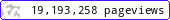•••••MadAsMaths.com :: Maths Booklets :: Further Topics :: Linear Algebra

FURTHER TOPICS - LINEAR ALGEBRA

These booklets are suitable for

• introduction/practice of vectors and matrices, for students taking A Level Mathematics or A Level Further Mathematics.

• introduction/revision/practice of vectors and matrices, for undergraduates in science degrees, science diplomas or mathematics degrees.

 consistency_of_equations.pdfmatrices_exam_questions_part_a.pdfmatrices_exam_questions_part_b.pdfmatrices_practice.pdfvector_exam_questions_part_a.pdfvector_practice_part_a.pdfvectors_exam_questions_part_b.pdfvectors_practice_part_b.pdf